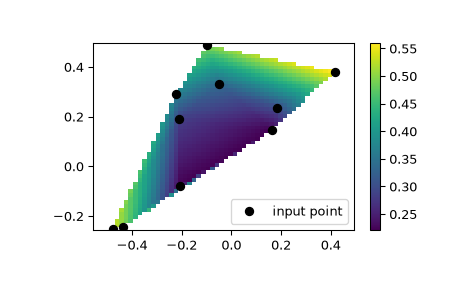# scipy.interpolate.LinearNDInterpolator¶

class scipy.interpolate.LinearNDInterpolator(points, values, fill_value=np.nan, rescale=False)

Piecewise linear interpolant in N dimensions.

New in version 0.9.

Parameters
pointsndarray of floats, shape (npoints, ndims); or Delaunay

Data point coordinates, or a precomputed Delaunay triangulation.

valuesndarray of float or complex, shape (npoints, …)

Data values.

fill_valuefloat, optional

Value used to fill in for requested points outside of the convex hull of the input points. If not provided, then the default is nan.

rescalebool, optional

Rescale points to unit cube before performing interpolation. This is useful if some of the input dimensions have incommensurable units and differ by many orders of magnitude.

griddata

Interpolate unstructured D-D data.

NearestNDInterpolator

Nearest-neighbor interpolation in N dimensions.

CloughTocher2DInterpolator

Piecewise cubic, C1 smooth, curvature-minimizing interpolant in 2D.

Notes

The interpolant is constructed by triangulating the input data with Qhull , and on each triangle performing linear barycentric interpolation.

References

1

http://www.qhull.org/

Examples

We can interpolate values on a 2D plane:

>>> from scipy.interpolate import LinearNDInterpolator
>>> import matplotlib.pyplot as plt
>>> np.random.seed(0)
>>> x = np.random.random(10) - 0.5
>>> y = np.random.random(10) - 0.5
>>> z = np.hypot(x, y)
>>> X = np.linspace(min(x), max(x))
>>> Y = np.linspace(min(y), max(y))
>>> X, Y = np.meshgrid(X, Y)  # 2D grid for interpolation
>>> interp = LinearNDInterpolator(list(zip(x, y)), z)
>>> Z = interp(X, Y)
>>> plt.plot(x, y, "ok", label="input point")
>>> plt.legend()
>>> plt.colorbar()
>>> plt.axis("equal")
>>> plt.show()Methods

 Evaluate interpolator at given points.

#### Previous topic

scipy.interpolate.griddata

#### Next topic

scipy.interpolate.LinearNDInterpolator.__call__